# Mind Map

## Area of parallelograms of Class 9

Let ABCD be any quadrilateral. Draw a diagonal AC. From B and D, draw perpendiculars BX and DY to AC.

Let AC = d, BX = p, DY = q

.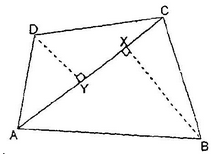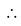Area of quadrilateral ABCD = Area of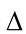ABC + Area ofADC

=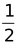AC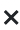BX +ACDY =dp +dq =d (p + q)

Ex:A quadrilateral ABCD is such that the diagonal BD divides its area into two equal parts.

Prove that BD bisects AC.

Sol: Let ABCD be a parallelogram having diagonals AC and BD. We have to prove that AC and BD bisect each other at O. i.e. AO = OC.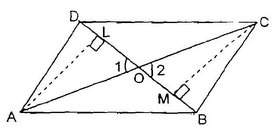Draw AL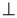BD and CMBD.

BD divides area ABCD into two equal parts.ar(ΔABD) = ar (ΔBDC) (given)

Thus, ΔABD and ΔBDC are on the same base AB are of equal area. Therefore, their, corresponding altitudes are equal.

i.e. AL = CM.

inΔALO and ΔCMO,

∠1 = ∠2 (vertically opposite angles)

∠ALO = ∠CMO (each equal to 90o)

and AL = CM

ΔALO  ≅  ΔCMO (AAS)

That means AO = OC (CPCT)BD bisects AC.

Ex: Find the area of quadrilateral ABCD (given √5 = 2.23)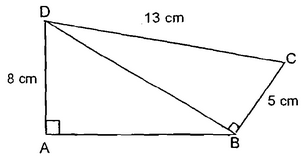Sol: In ΔBCD, CD2 = BD2 + BC2

⇒ (13)2 = (BD)2 + 52 ⇒  BD = 12

In ΔABD, BD2 = AB2 + AD2

⇒ (12)2 = (AB)2 + 82 ⇒  AB =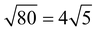ar(quad. ABCD) = ar(ΔABD) + ar(ΔBCD)

=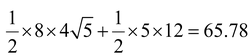cm2.

Ex: In the adjoining figure, AB || DC, DA is perpendicular to AB. Further DC = 7 cm,

CB = 10 cm, AB = 13 cm. Find the area of quadrilateral ABCD.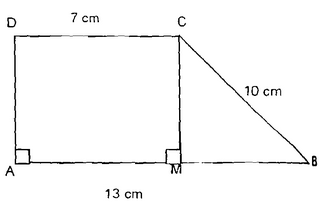Sol: Draw CMAB, then

AM = 7 cm, MB = 13 – 7 = 6 cm

BC = 10 cmfrom right triangle MBC

MC =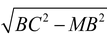(by Pythagoras theorem)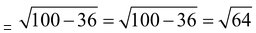= 8 cm.

Area of ABCD =(13 + 7) 8 = 80 cm2.

Ex: Show that the area of a rhombus is half the product of the lengths of its diagonals.

Sol: Let ABCD be a rhombus whose diagonals AC and BD intersect at O.

As the diagonals of a rhombus intersect at right anglesOBAC and ODAC.

Area (ABCD) = area (ΔABC) + area (ΔADC)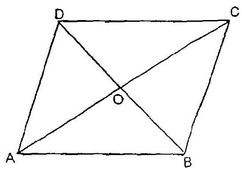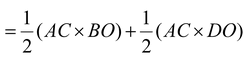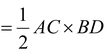.

Ex: If the diagonals AC and BD of a quadrilateral ABCD intersect at O and separate the quadrilateral ABCD into four triangle of equal area, show that the quadrilateral is a parallelogram.

Sol: Given: a quadrilateral ABCD, whose diagonals AC and BD intersect at O in such a way that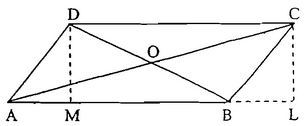ar(ΔAOB) = ar(ΔBOC)

= ar(ΔAOD) = ar(ΔCOD)

To prove: ABCD is a ||gm.

Construction: Draw CLAB and DMAB

Proof: ar(ΔAOD) = ar(ΔBOC)

⇒ar(ΔAOD) + ar(ΔAOB) = ar(ΔBOC) + ar(ΔAOB)

⇒ar(ΔABD) = ar(ΔABC)

⇒ DM = CL

⇒ DC || ABABCD is a parallelogram.

### Area of Trapezium:

ABCD is a trapezium in which AB || CD (AB < CD).

AL and BM are perpendiculars on CD from vertices A and B respectively.

Let AL = BM = h.

Area ABCD = Area of rectangle ABML + Area ofADL + Area ofBMC

=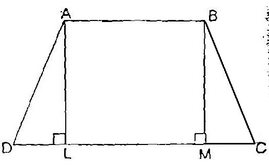=h(2AB + DL + MC) =h {AB + (LM + DL + MC)} (as AB = LM)

=h(AB + CD)Area of trapezium =×height(the sum of parallel sides) = h/2(a + b)

Where a = AB, b = CD, h = AL or BM.

Ex: Find the sum of the length of the bases of a trapezium whose area is 4.2 m2 and whose height is 280 cm.

Sol: Area = 4.2 m2

Height = 280 cm = 2.8 m

b1 + b2 = m.

Ex. If ABCD is a quadrilateral in which diagonals bisect at right angles and ACBD, then it is a

(a) Isosceles trapezium (b) Trapezium

(c) Rhombus (d) None

Sol. C

OD = OD, OA = OC

AOD = ∠COD = 90°

Similarly AD = BC = CD = AB

As digonals AC, BD are not equal, ABCD is a rhombus.

Important Types of Quadrilaterals Rectangles Squares Parallelograms Rhombus Trapezoid (trapezium) Kites The definitions are important.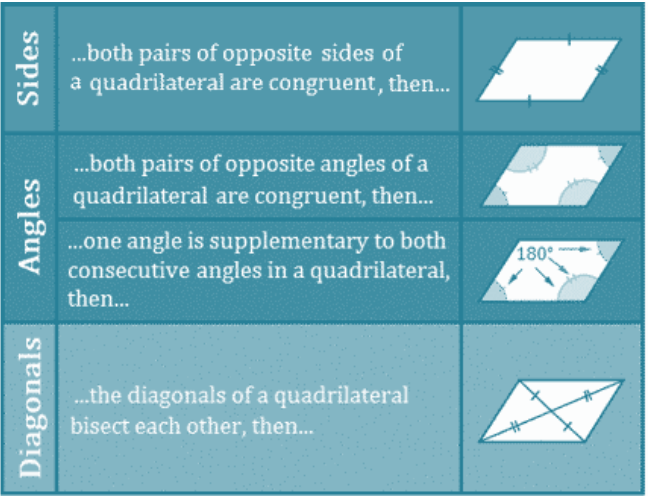### CONGRUENCE AREA AXIOM

•  If ΔABC≅ΔDEF

Then, ar. (ΔABC) = ar. (ΔDEF)

• Two Congruent figures have equal area but the Converse is not always true.
• Area of a parallelogram is the product of its base and the corresponding altitude.
• Area of a triangles is half the product of its base and the corresponding altitude.
• Triangles on the same base and between the same parallels are equal in area.
• A median of a triangle divides it into two triangles of equal area.